Anzeige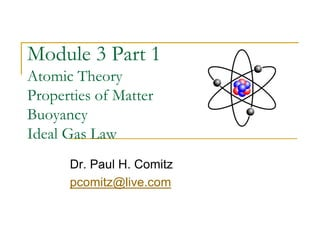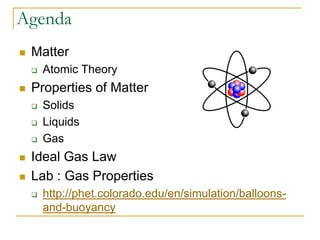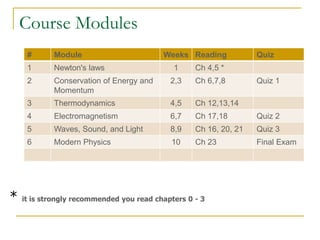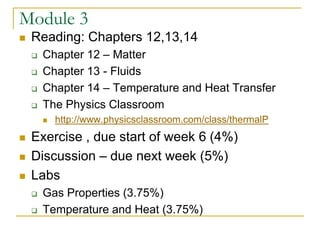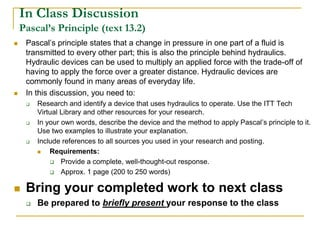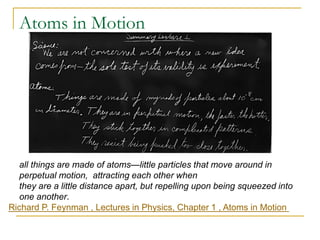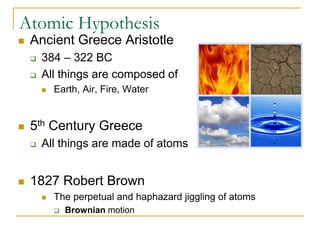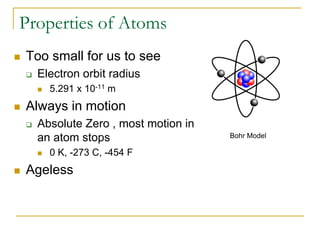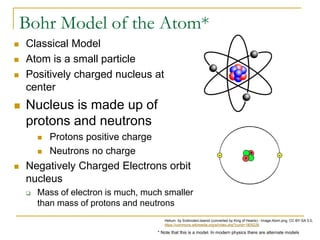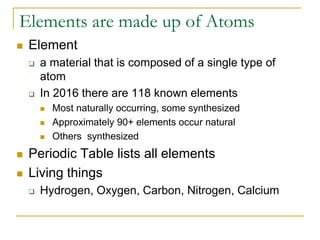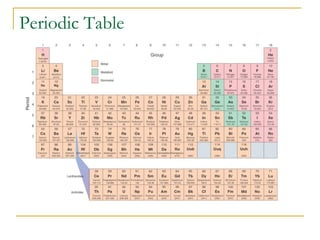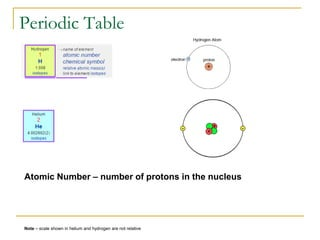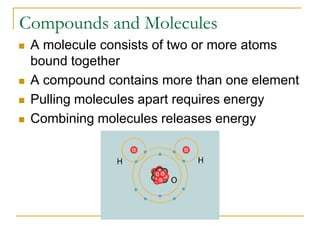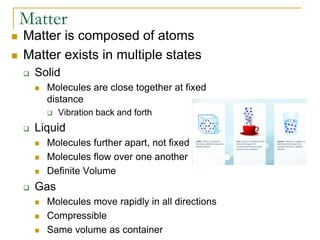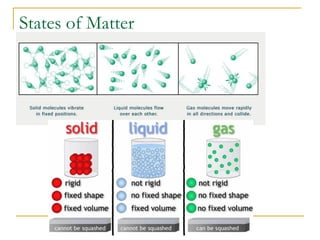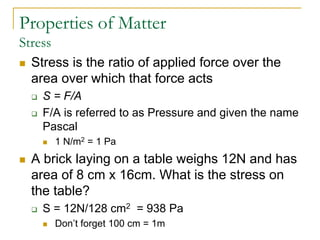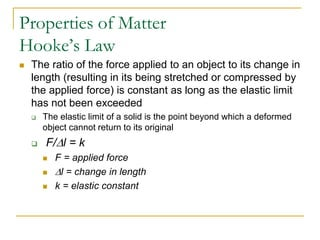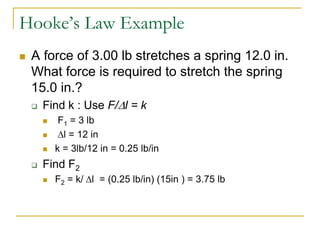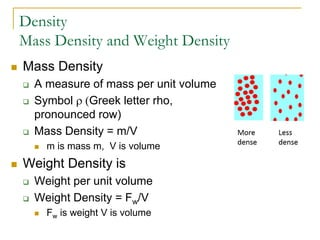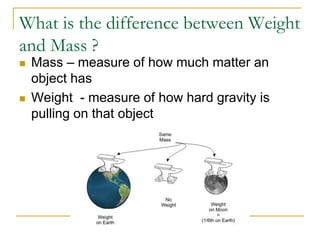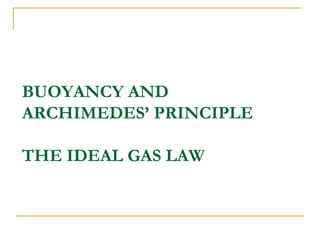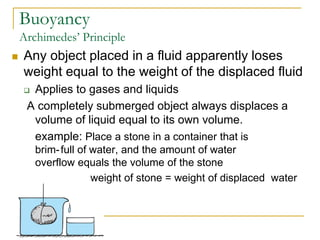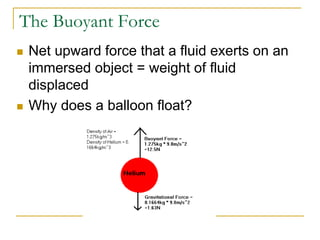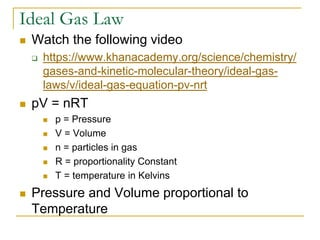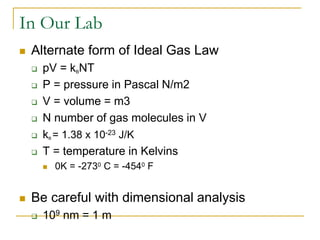1 von 25
Anzeige

### Physics Week 4 Atomic Theory

1. Module 3 Part 1 Atomic Theory Properties of Matter Buoyancy Ideal Gas Law Dr. Paul H. Comitz pcomitz@live.com
2. Agenda  Matter  Atomic Theory  Properties of Matter  Solids  Liquids  Gas  Ideal Gas Law  Lab : Gas Properties  http://phet.colorado.edu/en/simulation/balloons- and-buoyancy
3. Course Modules # Module Weeks Reading Quiz 1 Newton's laws 1 Ch 4,5 * 2 Conservation of Energy and Momentum 2,3 Ch 6,7,8 Quiz 1 3 Thermodynamics 4,5 Ch 12,13,14 4 Electromagnetism 6,7 Ch 17,18 Quiz 2 5 Waves, Sound, and Light 8,9 Ch 16, 20, 21 Quiz 3 6 Modern Physics 10 Ch 23 Final Exam * it is strongly recommended you read chapters 0 - 3
4. Module 3  Reading: Chapters 12,13,14  Chapter 12 – Matter  Chapter 13 - Fluids  Chapter 14 – Temperature and Heat Transfer  The Physics Classroom  http://www.physicsclassroom.com/class/thermalP  Exercise , due start of week 6 (4%)  Discussion – due next week (5%)  Labs  Gas Properties (3.75%)  Temperature and Heat (3.75%)
5. In Class Discussion Pascal’s Principle (text 13.2)  Pascal’s principle states that a change in pressure in one part of a fluid is transmitted to every other part; this is also the principle behind hydraulics. Hydraulic devices can be used to multiply an applied force with the trade-off of having to apply the force over a greater distance. Hydraulic devices are commonly found in many areas of everyday life.  In this discussion, you need to:  Research and identify a device that uses hydraulics to operate. Use the ITT Tech Virtual Library and other resources for your research.  In your own words, describe the device and the method to apply Pascal’s principle to it. Use two examples to illustrate your explanation.  Include references to all sources you used in your research and posting.  Requirements:  Provide a complete, well-thought-out response.  Approx. 1 page (200 to 250 words)  Bring your completed work to next class  Be prepared to briefly present your response to the class
6. Atoms in Motion Richard P. Feynman , Lectures in Physics, Chapter 1 , Atoms in Motion all things are made of atoms—little particles that move around in perpetual motion, attracting each other when they are a little distance apart, but repelling upon being squeezed into one another.
7. Atomic Hypothesis  Ancient Greece Aristotle  384 – 322 BC  All things are composed of  Earth, Air, Fire, Water  5th Century Greece  All things are made of atoms  1827 Robert Brown  The perpetual and haphazard jiggling of atoms  Brownian motion
8. Properties of Atoms  Too small for us to see  Electron orbit radius  5.291 x 10-11 m  Always in motion  Absolute Zero , most motion in an atom stops  0 K, -273 C, -454 F  Ageless Bohr Model
9. Bohr Model of the Atom*  Classical Model  Atom is a small particle  Positively charged nucleus at center  Nucleus is made up of protons and neutrons  Protons positive charge  Neutrons no charge  Negatively Charged Electrons orbit nucleus  Mass of electron is much, much smaller than mass of protons and neutrons Helium by Svdmolen/Jeanot (converted by King of Hearts) - Image:Atom.png, CC BY-SA 3.0, https://commons.wikimedia.org/w/index.php?curid=1805226 * Note that this is a model. In modern physics there are alternate models
10. Elements are made up of Atoms  Element  a material that is composed of a single type of atom  In 2016 there are 118 known elements  Most naturally occurring, some synthesized  Approximately 90+ elements occur natural  Others synthesized  Periodic Table lists all elements  Living things  Hydrogen, Oxygen, Carbon, Nitrogen, Calcium
11. Periodic Table
12. Periodic Table Note – scale shown in helium and hydrogen are not relative Atomic Number – number of protons in the nucleus
13. Compounds and Molecules  A molecule consists of two or more atoms bound together  A compound contains more than one element  Pulling molecules apart requires energy  Combining molecules releases energy
14. Matter  Matter is composed of atoms  Matter exists in multiple states  Solid  Molecules are close together at fixed distance  Vibration back and forth  Liquid  Molecules further apart, not fixed  Molecules flow over one another  Definite Volume  Gas  Molecules move rapidly in all directions  Compressible  Same volume as container
15. States of Matter
16. Properties of Matter Stress  Stress is the ratio of applied force over the area over which that force acts  S = F/A  F/A is referred to as Pressure and given the name Pascal  1 N/m2 = 1 Pa  A brick laying on a table weighs 12N and has area of 8 cm x 16cm. What is the stress on the table?  S = 12N/128 cm2 = 938 Pa  Don’t forget 100 cm = 1m
17. Properties of Matter Hooke’s Law  The ratio of the force applied to an object to its change in length (resulting in its being stretched or compressed by the applied force) is constant as long as the elastic limit has not been exceeded  The elastic limit of a solid is the point beyond which a deformed object cannot return to its original  F/Dl = k  F = applied force  Dl = change in length  k = elastic constant
18. Hooke’s Law Example  A force of 3.00 lb stretches a spring 12.0 in. What force is required to stretch the spring 15.0 in.?  Find k : Use F/Dl = k  F1 = 3 lb  Dl = 12 in  k = 3lb/12 in = 0.25 lb/in  Find F2  F2 = k/ Dl = (0.25 lb/in) (15in ) = 3.75 lb
19. Density Mass Density and Weight Density  Mass Density  A measure of mass per unit volume  Symbol r (Greek letter rho, pronounced row)  Mass Density = m/V  m is mass m, V is volume  Weight Density is  Weight per unit volume  Weight Density = Fw/V  Fw is weight V is volume
20. What is the difference between Weight and Mass ?  Mass – measure of how much matter an object has  Weight - measure of how hard gravity is pulling on that object
21. BUOYANCY AND ARCHIMEDES’ PRINCIPLE THE IDEAL GAS LAW
22. Buoyancy Archimedes’ Principle  Any object placed in a fluid apparently loses weight equal to the weight of the displaced fluid  Applies to gases and liquids A completely submerged object always displaces a volume of liquid equal to its own volume. example: Place a stone in a container that is brim-full of water, and the amount of water overflow equals the volume of the stone weight of stone = weight of displaced water
23. The Buoyant Force  Net upward force that a fluid exerts on an immersed object = weight of fluid displaced  Why does a balloon float?
24. Ideal Gas Law  Watch the following video  https://www.khanacademy.org/science/chemistry/ gases-and-kinetic-molecular-theory/ideal-gas- laws/v/ideal-gas-equation-pv-nrt  pV = nRT  p = Pressure  V = Volume  n = particles in gas  R = proportionality Constant  T = temperature in Kelvins  Pressure and Volume proportional to Temperature
25. In Our Lab  Alternate form of Ideal Gas Law  pV = kBNT  P = pressure in Pascal N/m2  V = volume = m3  N number of gas molecules in V  kB = 1.38 x 10-23 J/K  T = temperature in Kelvins  0K = -2730 C = -4540 F  Be careful with dimensional analysis  109 nm = 1 m
Anzeige